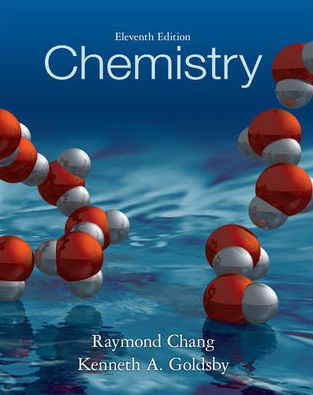×

×

Textbooks / Chemistry / Chemistry 11

# Chemistry 11th Edition - Solutions by Chapter## Full solutions for Chemistry | 11th Edition

ISBN: 9780073402680Chemistry | 11th Edition - Solutions by Chapter

Solutions by Chapter
4 5 0 368 Reviews
##### ISBN: 9780073402680

Since problems from 25 chapters in Chemistry have been answered, more than 1091474 students have viewed full step-by-step answer. This expansive textbook survival guide covers the following chapters: 25. Chemistry was written by and is associated to the ISBN: 9780073402680. The full step-by-step solution to problem in Chemistry were answered by , our top Chemistry solution expert on 11/08/17, 03:59AM. This textbook survival guide was created for the textbook: Chemistry, edition: 11.

Key Chemistry Terms and definitions covered in this textbook
• Alkyl group

A group derived by removing a hydrogen from an alkane; given the symbol R!

• atomic weight

The average mass of the atoms of an element in atomic mass units (amu); it is numerically equal to the mass in grams of one mole of the element. (Section 2.4)

• Bimolecular reaction

A reaction in which two species are involved in the rate-determining step.

• bond enthalpy.

The enthalpy change required to break a bond in a mole of gaseous molecules. (9.10)

• core electrons.

All nonvalence electrons in an atom. (8.2)

• diatomic molecule

A molecule composed of only two atoms. (Section 2.6)

• diene

A compound containing two carbon-carbon p bonds.

• Endergonic reaction

A reaction in which the Gibbs free energy of the products is higher than that of the reactants. The position of equilibrium for an endergonic reaction favors starting materials

• glucose

A polyhydroxy aldehyde whose formula is CH2OH1CHOH24CHO; it is the most important of the monosaccharides. (Section 24.8)

• Hyperconjugation

Interaction of electrons in a s-bonding orbital with the vacant 2p orbital of an adjacent positively charged carbon.

• intermediate

A structure corresponding to a local minimum (valley) in an energy diagram.

• Ka

A measure of the strength of an acid: Ka = Keq 3H2O4 = 3H3O+ 4 3A- 4 3HA4

• Ketone

A compound containing a carbonyl group bonded to two carbons.

• LUMO

The lowest unoccupied molecular orbital.

• Messenger RNA (mRNA)

A ribonucleic acid that carries coded genetic information from DNA to the ribosomes for the synthesis of proteins

• nonelectrolyte

A substance that does not ionize in water and consequently gives a nonconducting solution. (Section 4.1)

• partial pressure

The pressure exerted by a particular gas in a mixture. (Section 10.6)

• polar covalent bond

A covalent bond in which the electrons are not shared equally. (Section 8.4)

• reactant

A starting substance in a chemical reaction; it appears to the left of the arrow in a chemical equation. (Section 3.1)

• root-mean-square (rms) speed 1M2

The square root of the average of the squared speeds of the gas molecules in a gas sample. (Section 10.7)